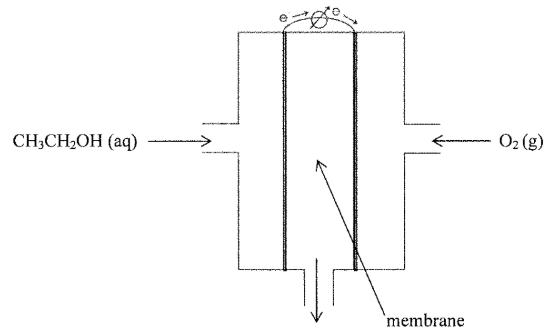# Problem: An illustration of an ethanol fuel cell is given below:The half reactions (written as reductions) for the fuel cell at 25 °C are given below.O2 (g) + 4 H3O+ (aq) + 4 e- → 6 H2O (l)                                                        ε°red = 1.23 VCH3COOH (aq) + 4 H3O+ (aq) + 4 e- → CH3CH2OH (aq) + 5 H2O (l)         ε°red = ???   acetic acid                                                 ethanolCalculate the half-cell reduction potential for the anode at 25 °C. Please circle your answer.

###### FREE Expert Solution

We are asked to half-cell reduction potential for the anode at 25 °C.

Recall:

where:

n = number of moles electrons

F = Faraday’s constant, 96485 C/mol e-

ε° = standard reduction potential

We can use the following equation to solve for ΔG˚rxn:

We go through the following steps to solve the problem:

Step 1: Write the overall chemical reaction

Step 2: Calculate  ΔG°rxn.

Step 3: Calculate the overall potential for the fuel cell

Step 4: Calculate half-cell reduction potential for the anode at 25 °C

Step 1: Write the overall chemical reaction

Recall the mnemonics LEO GER.

Lose               Gain

Electron         Electrons

Oxidation       Reduction

82% (139 ratings)###### Problem Details

An illustration of an ethanol fuel cell is given below:The half reactions (written as reductions) for the fuel cell at 25 °C are given below.

O2 (g) + 4 H3O+ (aq) + 4 e- → 6 H2O (l)                                                        ε°red = 1.23 V

CH3COOH (aq) + 4 H3O+ (aq) + 4 e- → CH3CH2OH (aq) + 5 H2O (l)         ε°red = ???

acetic acid                                                 ethanol